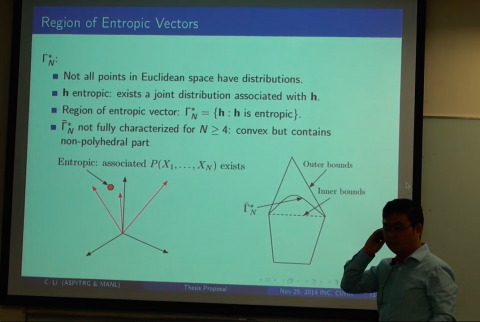# Multi-source Multi-sink Networks: Rate Regions, Codes, Computations, & Forbidden MinorsMr. Congduan LI
ECE Department, Drexel University
Date:
Tuesday, 25 November, 2014
Time:
11:00 am – 12:00 pm
Venue:
Room 833, Ho Sin Hang Engineering Building
Abstract:

In this talk, the rate regions of multi-source multi-sink networks are investigated.  The topics include sufficiency of simple linear codes, computations of rate regions, and network operations preserving insufficiency of linear codes.  As a special class of multi-source multi-sink networks, multilevel diversity coding systems (MDCS) are investigated as a demonstration.

After introducing rate region problems in multi-terminal networks, the Shannon outer bound and several achievable inner bounds based on representable matroids or linear codes are given for each non-isomorphic instance.  For thousands of MDCS instances, the bounds match, and hence exact rate regions are proven. Results gained from these computations are summarized in key statistics involving aspects such as the sufficiency of scalar binary codes, the necessary size of vector binary codes, etc. Also, it is shown how to generate computer aided human readable converse proofs, as well as how to construct the codes for an achievability proof.

Based on this large repository of rate regions for all small MDCS networks, a series of results about general large MDCS networks that they inspired are introduced and proved. In particular, in order to scale small network studies to networks of arbitrary size, a series of embedding operations that preserve the property of sufficiency of scalar or vector codes are presented. The utility of these operations is demonstrated by boiling the thousands of MDCS instances for which binary scalar codes are insufficient down to 12 forbidden smallest embedded MDCS instances.

The proposed directions for future study focus on: 1) the scaling of code class sufficiency results to networks of arbitrary size, 2) applications of the techniques developed to asymmetric distributed storage problems, 3) the interplay between the network embedding operations and the need for non-Shannon inequalities in determining a rate region, and 4) an exhaustive theoretical characterization of extremal polymatroids — extreme rays of the Shannon outer bound.

Biography:

Congduan Li is currently a Ph.D. candidate in ECE Department, Drexel University. He received his BS and MS degrees in EE from University of Science and Technology Beijing and Northern Arizona University, respectively. He is doing research under the guidance of Dr. John Walsh and Dr. Steven Weber. His current research focuses on developing effective algorithms and software to use computers to prove rate regions for general multi-terminal networks and characterizing properties of networks for which simple linear codes suffice for the entire rate regions.

Announcement:sem2314_INC_Congduan LI_WHY251114.pdf